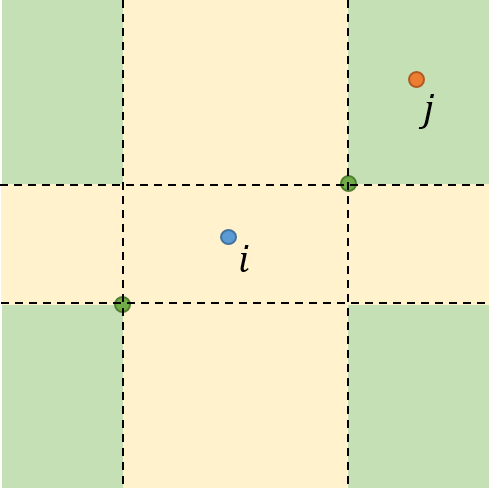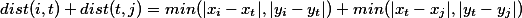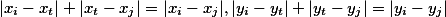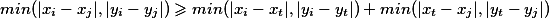题目大意解题思路1. 首先对所有点的x的坐标进行排序
2. 遍历排序后的x点坐标，若有连续一段的x值都相等，则我们将这一段中所有点都与第一个点(将这个点称为代表点)连边，距离为0

``````sort(x)
i = 1
While (i ≤ n) Then
j = i + 1
While (j ≤ n and x[i].val == x[j].val) Then
连接边(x[i].id, x[j].id, 0)    // 因为无向图，所以需要连接两条边
j = j + 1
End If
i = j
End While
````````````sort(x)
i = 1
While (i ≤ n) Then
j = i + 1
While (j ≤ n and x[i].val == x[j].val) Then
j = j + 1
End If
If (j ≤ n) Then
连接边(x[i].id, x[j].id, x[j].val - x[i].val)
End
End While
``````

• 邻居节点不是很理解；如果它前提是x,y值都不相等的话，那黄色区域中可以有其它点，比如某点和i是同x轴的；而如果它前提是x，y值有一个不同就可以，那某点和i是x相等的就是邻居节点，这时候它可以大于4。所以三角定理是不成立的

• 添加评论

• 而且Dijstra已经用了优先队列优化。

• 好吧ac了。。 原来是我预处理用的list，排序比较慢吧

• 添加评论